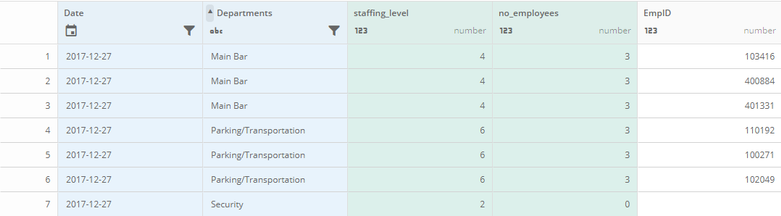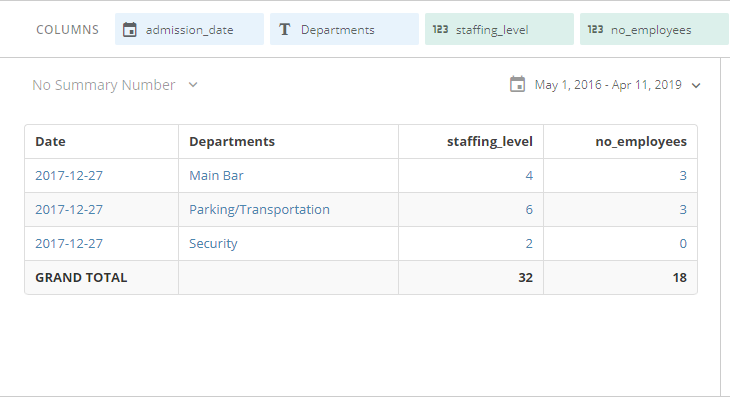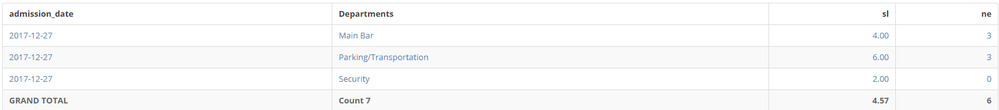# Table Card Total Rows Incorrect

I am trying to create a table card summarizing this data by Date and Department:Summarized the table looks like the following:How can I get the total rows to be correct on the card? I want the ability for the user to be able to drill down into a department and see a list of employees.

• Ahh, I see what is happening here. You have duplicates in your dataset because of the employee id. You won't be able to achieve the result using total row as that is going to either sum or count the fields you have inputted. You will need to create a row in your dataset where you do the aggregation for grand total using a MySQL dataflow or Magic ETL.

You would aggregate it by date and put the "grand total" text under the department field and sum the max values of each department for a specific date, then union it to your final dataset.

Thanks,

Brian

• I'll take a stab at this one...

Try creating new beast modes to calculate staffing level and number of employees

Staffing Level:

sum(staffing_level) / count(distinct ifnull(EmpID,1))

Number of Employees:

count(distinct ifnull(EmpID,0))

Then use these fields in your table instead of the current fields you are using.

You should then still be able to drill down and see EmpID if needed

• Hello,

I think you need to change your calculation to sum or count for staffing_level and no_employees by clicking the little pencil button when you hover.

Hope this helps,

Brian

• Brian,

I could create and add total rows to the dataflow but then my card has no dynamic capabilities and any card filtering at the department level will not be reflected in the totals.

Originally this card was summarized at the daily level and subtotals/totals worked fine but users are requesting the ability to drill down into the detail of each department and view employees in that total.

Any recommendations how I can summarize the card without losing the ability to drill down to the details?

Thank you,

Chris

• Getting closer but still a strange total on the staffing_level beast mode.Here are the beast modes I am using

staffing_level

sum(`staffing_level`)/count(distinct ifnull(`EmpID`,1))

number_employees

count(distinct `EmpID`)

**  I had to remove the ifnull() because it was causing null records to be counted as 1 **

• You could try this ... the only issue will be is if two department staffing levels are the same, then it will calculate incorrectly.

sum(distinct `staffing_level`)/count(distinct `EmpID`)

Thanks,

Brian

• Another alternative would be a window function. I have never actually tried to use this in beastmode but it would look something like ...

`CASE WHEN `EmpID` IS NULLTHEN staffing_levelELSE SUM(distinct staffing_level) OVER (PARTITION BY department)/count(distinct `EmpID`)END`

Thanks,

Brian

• Wait a second,

Why are we trying to divide staffing level? Is it just supposed to be a sum of total employees needed?

in this scenario, you can just do SUM(DISTINCT staffing_level)

Thanks,

Brian

• Brian,

sum(distinct `staffing_level`) will not work correctly if two departments have the same staffing level.  We are attempting to sum all rows and divide it by the number of employees.  This works for each row but the grand total row for some reason is not a whole number.

In this example using the Main Bar department the sum(`staffing_level`) = 12 and count(distinct `empID`) = 3 and 12/3=4 which is the number I expect. This does not explain why the total row is incorrect on the table where it should be adding 4+6+2 = 12 but is displaying 4.57??

• The total row is just completing whichever calculation you give it on an aggregate level. So when you have sum(staffing_level) / count(distinct ifnull(empID,1)), your total row is doing 4+4+4+6+6+6+2 = 32 / 7 = 4.57.

• This is why in one of my first posts I mentioned you may want to create an "All Corporate" department, which has the totals already calculated. You can then put together a table like the attached image using MAX(staffing_level) and MAX(no_employees) as your two fields.

Hope this helps,

Brian

• It is important that the totals remain calculated on the fly due to PDP policies and applying card filters.  I was able to create the card with the correct totals and the drill down functionality by editing my dataflow to stage the data differently so I could sum all of the fields.

Thank you all for the feedback and ideas.  I love to use the Dojo as a sounding board when I get stuck!

•

Can you make the replies that best helped you by clicking on Accept as Solution so others can benefit from this thread.

Love the collaboration! NIce work @Property_Ninja and @ST_-Superman-_!

Regards,

• I marked solutions by both @Property_Ninja and @ST_-Superman-_ as they both helped me in arriving at the final solution.

Thanks again.

• Thank you!

This discussion has been closed.